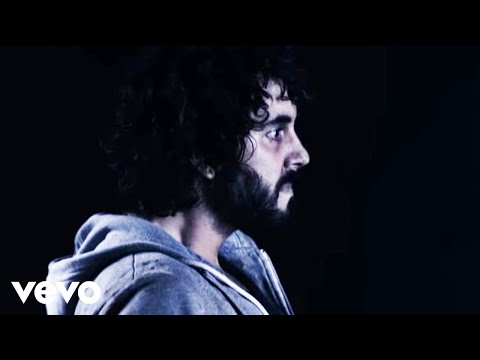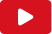# Testo Here We Go

## Testo Here We Go

(M. Cooper, B. Higgins, M. Gray, L. Nystrom)

Put your tongue in my ear
It's queer but kinda fun
We're the tomorrow generation
There's much to be done
Step this way
The modern girl's delighted to
Be risqué

Here we go
We're gonna rock 'n' roll till stop
And them we'll drop
It's a squeeze
But if you just say please
Then that's fine
It'll be sublime
The bass line cuts deeper than
The sharpest stiletto
So one, two, three, now baby
Here we go
Here we go, here we go
You're my Romeo
Here we go, here we go
Geek or unique, hell
Get on with the show
Here we go, here we go
You're my hero
Here we go, here we go, here we go

Sugar, give me your face and
Place it next to mine
'Cause darling, grease is the word
And boy, the word's divine
I don't do sex, but I do do
Second base
So I suggest you wipe that frown

Here we go
We're gonna rock 'n' roll till we stop (stop)
And then we'll drop
It's a squeeze
But if you just say please
Then that's fine (fine)
It'll be sublime
The bass line cuts deeper than
The sharpest stiletto
So, one two, three, now baby
Here we go

Here we go, here we go
You're my Romeo
Here we go, here we go
Geek or unique, hell
Get on with the show
Here we go, here we go
You're my hero
Here we go, here we go, here we g,g,g,g,g,go

Here we go, here we go
You're my Romeo
Here we go, here we go
Geek or unique, hell
Get on with the show
Here we go, here we go
You're my hero
Here we go, here we go, here we g,g,g,g,g,go

Here we go, here we go